## Matplotlib 数据可视化基础教程

6438 字 · 2498 阅读 · 2019 年 06 月 04 日%matplotlib inline


### 简单图形绘制

from matplotlib import pyplot as plt


plt.plot([1, 2, 3, 2, 1, 2, 3, 4, 5, 6, 5, 4, 3, 2, 1])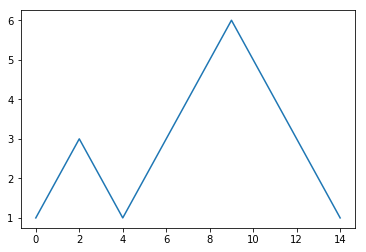plt.plot()pyplot 模块下面的直线绘制（折线图）方法类。示例中包含了一个 [1, 2, 3, 2, 1, 2, 3, 4, 5, 6, 5, 4, 3, 2, 1] 列表，Matplotlib 会默认将该列表作为 $y$ 值，而 $x$ 值会从 $0$ 开始依次递增。

plt.plot([2, 3, 4, 5, 6, 7, 8, 9, 10, 11, 12, 13, 14, 15, 16],
[1, 2, 3, 2, 1, 2, 3, 4, 5, 6, 5, 4, 3, 2, 1])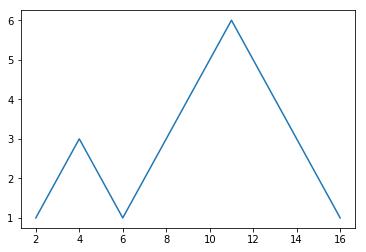pyplot 模块中 pyplot.plot 方法是用来绘制折线图的。你应该会很容易联想到，更改后面的方法类名就可以更改图形的样式。的确，在 Matplotlib 中，大部分图形样式的绘制方法都存在于 pyplot 模块中。例如：

matplotlib.pyplot.angle_spectrum 绘制电子波谱图
matplotlib.pyplot.bar 绘制柱状图
matplotlib.pyplot.barh 绘制直方图
matplotlib.pyplot.broken_barh 绘制水平直方图
matplotlib.pyplot.contour 绘制等高线图
matplotlib.pyplot.errorbar 绘制误差线
matplotlib.pyplot.hexbin 绘制六边形图案
matplotlib.pyplot.hist 绘制柱形图
matplotlib.pyplot.hist2d 绘制水平柱状图
matplotlib.pyplot.pie 绘制饼状图
matplotlib.pyplot.quiver 绘制量场图
matplotlib.pyplot.scatter 散点图
matplotlib.pyplot.specgram 绘制光谱图

matplotlib.pyplot.plot(*args, **kwargs) 方法严格来讲可以绘制线形图或者样本标记。其中，*args 允许输入单个 $y$ 值或 $x, y$ 值。

import numpy as np  # 载入数值计算模块

# 在 -2PI 和 2PI 之间等间距生成 1000 个值，也就是 X 坐标
X = np.linspace(-2*np.pi, 2*np.pi, 1000)
# 计算 y 坐标
y = np.sin(X)

# 向方法中 *args 输入 X，y 坐标
plt.plot(X, y)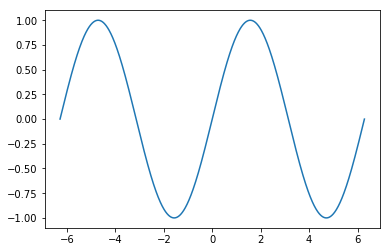plt.bar([1, 2, 3], [1, 2, 3])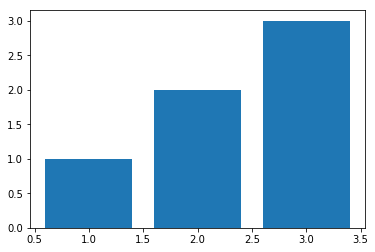# X,y 的坐标均有 numpy 在 0 到 1 中随机生成 1000 个值
X = np.random.ranf(1000)
y = np.random.ranf(1000)
# 向方法中 *args 输入 X，y 坐标
plt.scatter(X, y)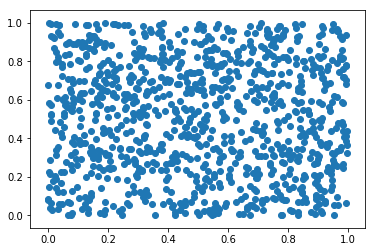plt.pie([1, 2, 3, 4, 5])X, y = np.mgrid[0:10, 0:10]
plt.quiver(X, y)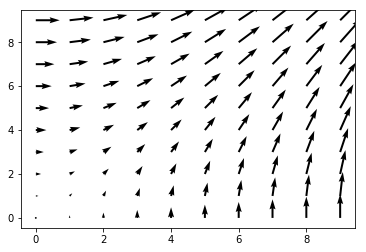# 生成网格矩阵
x = np.linspace(-5, 5, 500)
y = np.linspace(-5, 5, 500)
X, Y = np.meshgrid(x, y)
# 等高线计算公式
Z = (1 - X / 2 + X ** 3 + Y ** 4) * np.exp(-X ** 2 - Y ** 2)

plt.contourf(X, Y, Z)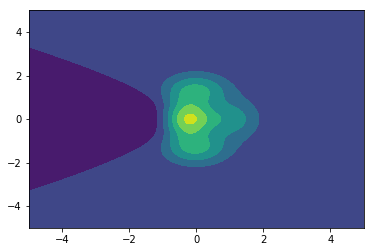### 定义图形样式

alpha= 设置线型的透明度，从 0.0 到 1.0
color= 设置线型的颜色
fillstyle= 设置线型的填充样式
linestyle= 设置线型的样式
linewidth= 设置线型的宽度
marker= 设置标记点的样式
…… ……

# 在 -2PI 和 2PI 之间等间距生成 1000 个值，也就是 X 坐标
X = np.linspace(-2 * np.pi, 2 * np.pi, 1000)
# 计算 sin() 对应的纵坐标
y1 = np.sin(X)
# 计算 cos() 对应的纵坐标
y2 = np.cos(X)

# 向方法中 *args 输入 X，y 坐标
plt.plot(X, y1, color='r', linestyle='--', linewidth=2, alpha=0.8)
plt.plot(X, y2, color='b', linestyle='-', linewidth=2)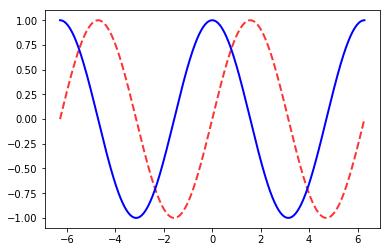s= 散点大小
c= 散点颜色
marker= 散点样式
cmap= 定义多类别散点的颜色
alpha= 点的透明度
edgecolors= 散点边缘颜色
# 生成随机数据
x = np.random.rand(100)
y = np.random.rand(100)
colors = np.random.rand(100)
size = np.random.normal(50, 60, 10)

plt.scatter(x, y, s=size, c=colors)  # 绘制散点图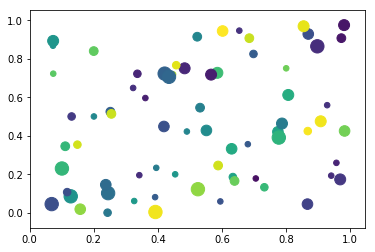label = 'Cat', 'Dog', 'Cattle', 'Sheep', 'Horse'  # 各类别标签
color = 'r', 'g', 'r', 'g', 'y'  # 各类别颜色
size = [1, 2, 3, 4, 5]  # 各类别占比
explode = (0, 0, 0, 0, 0.2)  # 各类别的偏移半径
# 绘制饼状图
plt.pie(size, colors=color, explode=explode,
# 饼状图呈正圆
plt.axis('equal')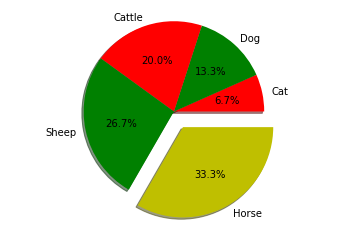### 组合图形样式

x = [1, 3, 5, 7, 9, 11, 13, 15, 17, 19]
y_bar = [3, 4, 6, 8, 9, 10, 9, 11, 7, 8]
y_line = [2, 3, 5, 7, 8, 9, 8, 10, 6, 7]

plt.bar(x, y_bar)
plt.plot(x, y_line, '-o', color='y')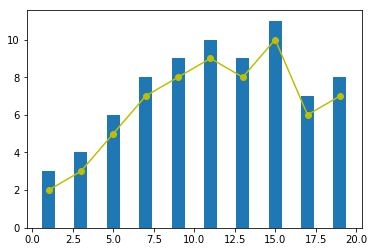### 定义图形位置

x = np.linspace(0, 10, 20)  # 生成数据
y = x * x + 2

fig = plt.figure()  # 新建图形对象
axes = fig.add_axes([0.5, 0.5, 0.8, 0.8])  # 控制画布的左，下，宽度，高度
axes.plot(x, y, 'r')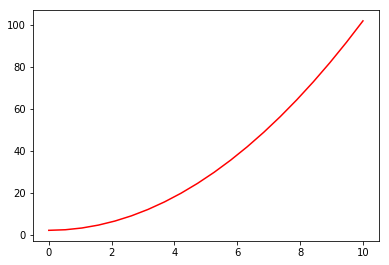fig = plt.figure()  # 新建画板
axes1 = fig.add_axes([0.1, 0.1, 0.8, 0.8])  # 大画布
axes2 = fig.add_axes([0.2, 0.5, 0.4, 0.3])  # 小画布

axes1.plot(x, y, 'r')  # 大画布
axes2.plot(y, x, 'g')  # 小画布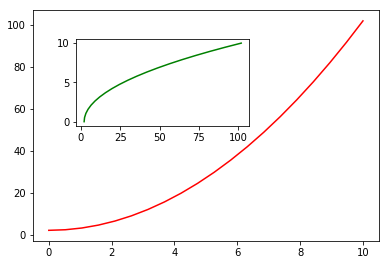fig, axes = plt.subplots()
axes.plot(x, y, 'r')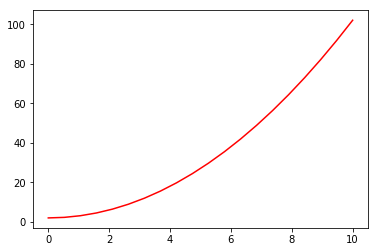fig, axes = plt.subplots(nrows=1, ncols=2)  # 子图为 1 行，2 列
for ax in axes:
ax.plot(x, y, 'r')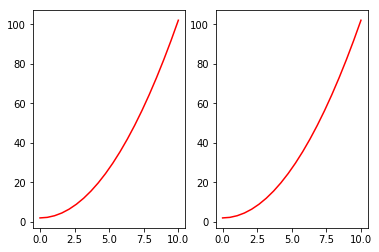fig, axes = plt.subplots(
figsize=(16, 9), dpi=50)  # 通过 figsize 调节尺寸，dpi 调节显示精度
axes.plot(x, y, 'r')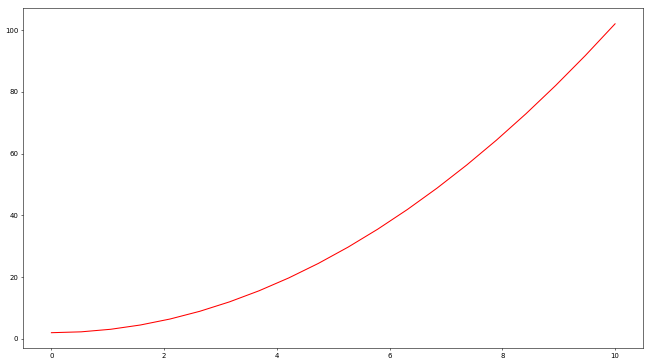### 规范绘图方法

#### 添加图标题、图例

fig, axes = plt.subplots()

axes.set_xlabel('x label')  # 横轴名称
axes.set_ylabel('y label')
axes.set_title('title')  # 图形名称

axes.plot(x, x**2)
axes.plot(x, x**3)
axes.legend(["y = x**2", "y = x**3"], loc=0)  # 图例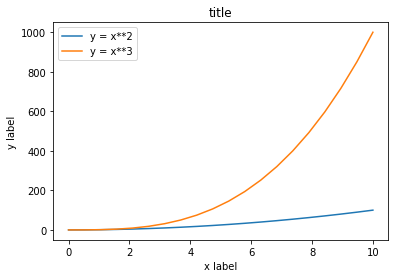#### 线型、颜色、透明度

fig, axes = plt.subplots()

axes.plot(x, x+1, color="red", alpha=0.5)
axes.plot(x, x+2, color="#1155dd")
axes.plot(x, x+3, color="#15cc55")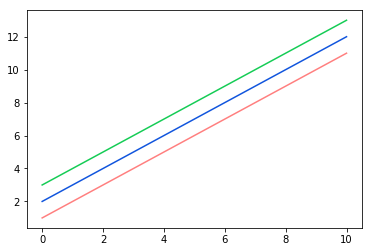fig, ax = plt.subplots(figsize=(12, 6))

# 线宽
ax.plot(x, x+1, color="blue", linewidth=0.25)
ax.plot(x, x+2, color="blue", linewidth=0.50)
ax.plot(x, x+3, color="blue", linewidth=1.00)
ax.plot(x, x+4, color="blue", linewidth=2.00)

# 虚线类型
ax.plot(x, x+5, color="red", lw=2, linestyle='-')
ax.plot(x, x+6, color="red", lw=2, ls='-.')
ax.plot(x, x+7, color="red", lw=2, ls=':')

# 虚线交错宽度
line, = ax.plot(x, x+8, color="black", lw=1.50)
line.set_dashes([5, 10, 15, 10])

# 符号
ax.plot(x, x + 9, color="green", lw=2, ls='--', marker='+')
ax.plot(x, x+10, color="green", lw=2, ls='--', marker='o')
ax.plot(x, x+11, color="green", lw=2, ls='--', marker='s')
ax.plot(x, x+12, color="green", lw=2, ls='--', marker='1')

# 符号大小和颜色
ax.plot(x, x+13, color="purple", lw=1, ls='-', marker='o', markersize=2)
ax.plot(x, x+14, color="purple", lw=1, ls='-', marker='o', markersize=4)
ax.plot(x, x+15, color="purple", lw=1, ls='-',
marker='o', markersize=8, markerfacecolor="red")
ax.plot(x, x+16, color="purple", lw=1, ls='-', marker='s', markersize=8,
markerfacecolor="yellow", markeredgewidth=2, markeredgecolor="blue")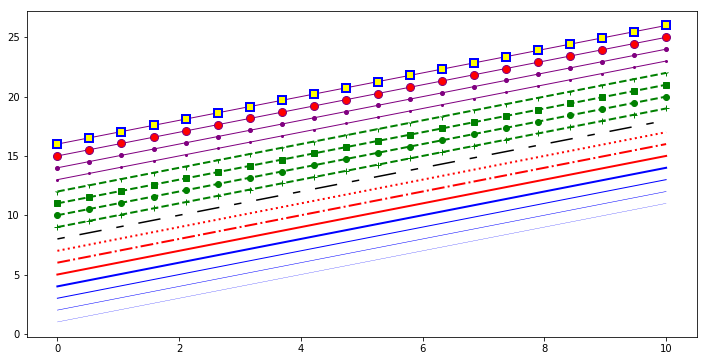#### 画布网格、坐标轴范围

fig, axes = plt.subplots(1, 2, figsize=(10, 5))

# 显示网格
axes.plot(x, x**2, x, x**3, lw=2)
axes.grid(True)

# 设置坐标轴范围
axes.plot(x, x**2, x, x**3)
axes.set_ylim([0, 60])
axes.set_xlim([2, 5])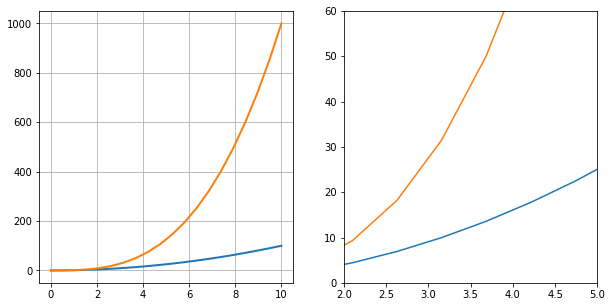n = np.array([0, 1, 2, 3, 4, 5])

fig, axes = plt.subplots(1, 4, figsize=(16, 5))

axes.scatter(x, x + 0.25*np.random.randn(len(x)))
axes.set_title("scatter")

axes.step(n, n**2, lw=2)
axes.set_title("step")

axes.bar(n, n**2, align="center", width=0.5, alpha=0.5)
axes.set_title("bar")

axes.fill_between(x, x**2, x**3, color="green", alpha=0.5)
axes.set_title("fill_between")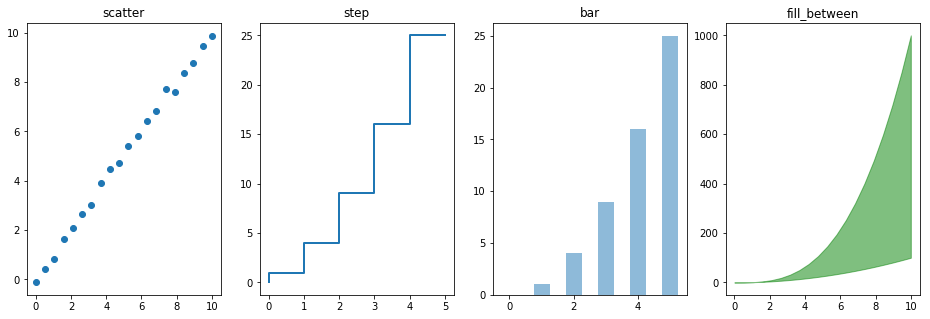### 图形标注方法

Matplotlib 中，文字标注的方法由 matplotlib.pyplot.text() 实现。最基本的样式为 matplotlib.pyplot.text(x, y, s)，其中 x, y 用于标注位置定位，s 代表标注的字符串。除此之外，你还可以通过 fontsize= , horizontalalignment= 等参数调整标注字体的大小，对齐样式等。

fig, axes = plt.subplots()

x_bar = [10, 20, 30, 40, 50]  # 柱形图横坐标
y_bar = [0.5, 0.6, 0.3, 0.4, 0.8]  # 柱形图纵坐标
bars = axes.bar(x_bar, y_bar, color='blue', label=x_bar, width=2)  # 绘制柱形图
for i, rect in enumerate(bars):
x_text = rect.get_x()  # 获取柱形图横坐标
y_text = rect.get_height() + 0.01  # 获取柱子的高度并增加 0.01
plt.text(x_text, y_text, '%.1f' % y_bar[i])  # 标注文字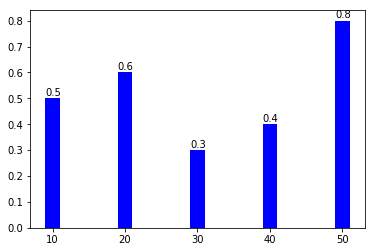fig, axes = plt.subplots()

bars = axes.bar(x_bar, y_bar, color='blue', label=x_bar, width=2)  # 绘制柱形图
for i, rect in enumerate(bars):
x_text = rect.get_x()  # 获取柱形图横坐标
y_text = rect.get_height() + 0.01  # 获取柱子的高度并增加 0.01
plt.text(x_text, y_text, '%.1f' % y_bar[i])  # 标注文字

# 增加箭头标注
plt.annotate('Min', xy=(32, 0.3), xytext=(36, 0.3),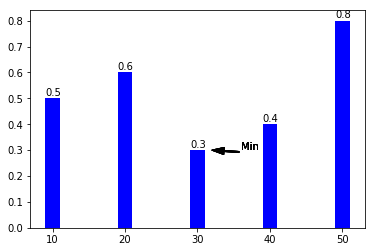### 兼容 MATLAB 代码风格接口

：本部分内容适合于之前有 MATLAB 基础的用户了解，其他读者可以直接跳过。

from matplotlib import pylab


x = np.linspace(0, 10, 20)
y = x * x + 2


pylab.plot(x, y, 'r')  # 'r' 代表 redpylab.subplot(1, 2, 1)  # 括号中内容代表（行，列，索引）
pylab.plot(x, y, 'r--')  # ‘’ 中的内容确定了颜色和线型

pylab.subplot(1, 2, 2)
pylab.plot(y, x, 'g*-')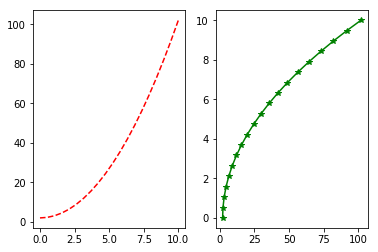### 三维图形绘制

mplot3d 模块下主要包含 4 个大类，分别是：

• mpl_toolkits.mplot3d.axes3d()
• mpl_toolkits.mplot3d.axis3d()
• mpl_toolkits.mplot3d.art3d()
• mpl_toolkits.mplot3d.proj3d()

import numpy as np
from mpl_toolkits.mplot3d import Axes3D
import matplotlib.pyplot as plt
%matplotlib inline

# x, y, z 均为 0 到 1 之间的 100 个随机数
x = np.random.normal(0, 1, 100)
y = np.random.normal(0, 1, 100)
z = np.random.normal(0, 1, 100)

fig = plt.figure()

ax = Axes3D(fig)
ax.scatter(x, y, z)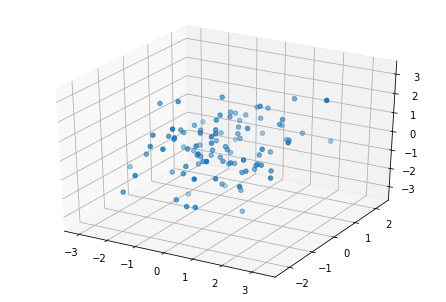# 生成数据
x = np.linspace(-6 * np.pi, 6 * np.pi, 1000)
y = np.sin(x)
z = np.cos(x)

# 创建 3D 图形对象
fig = plt.figure()
ax = Axes3D(fig)
ax.plot(x, y, z)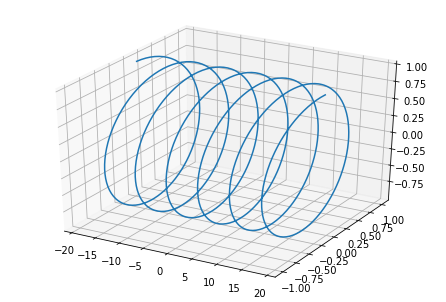# 创建 3D 图形对象
fig = plt.figure()
ax = Axes3D(fig)

# 生成数据并绘图
x = [0, 1, 2, 3, 4, 5, 6]
for i in x:
y = [0, 1, 2, 3, 4, 5, 6, 7, 8, 9]
z = abs(np.random.normal(1, 10, 10))
ax.bar(y, z, i, zdir='y', color=['r', 'g', 'b', 'y'])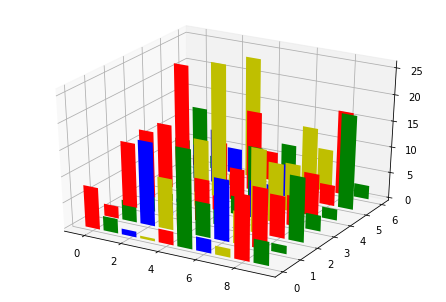# 创建 3D 图形对象
fig = plt.figure()
ax = Axes3D(fig)

# 生成数据
X = np.arange(-2, 2, 0.1)
Y = np.arange(-2, 2, 0.1)
X, Y = np.meshgrid(X, Y)
Z = np.sqrt(X ** 2 + Y ** 2)

# 绘制曲面图，并使用 cmap 着色
ax.plot_surface(X, Y, Z, cmap=plt.cm.winter)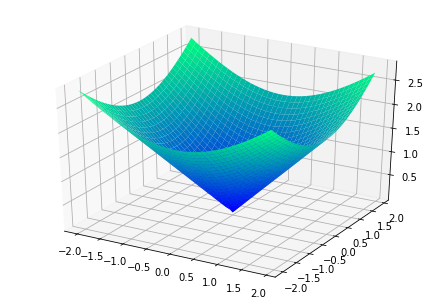cmap=plt.cm.winter 表示采用了 winter 配色方案。除了通过 Axes3D() 声明三维图形，我们也可以通过 projection='3d' 参数声明 3D 图形。

fig = plt.figure(figsize=(14, 6))

# 通过 projection='3d' 声明绘制 3D 图形
ax = fig.add_subplot(1, 2, 1, projection='3d')
ax.plot_surface(X, Y, Z, cmap=plt.cm.winter)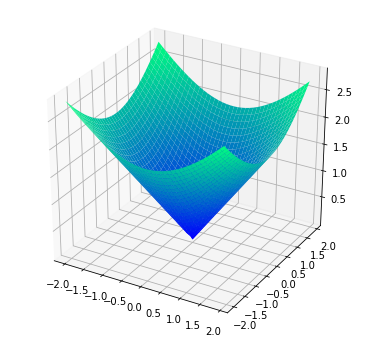### 三维混合图

# 创建 3D 图形对象
fig = plt.figure()
ax = Axes3D(fig)

# 生成数据并绘制图 1
x1 = np.linspace(-3 * np.pi, 3 * np.pi, 500)
y1 = np.sin(x1)
ax.plot(x1, y1, zs=0, c='red')

# 生成数据并绘制图 2
x2 = np.random.normal(0, 1, 100)
y2 = np.random.normal(0, 1, 100)
z2 = np.random.normal(0, 1, 100)
ax.scatter(x2, y2, z2)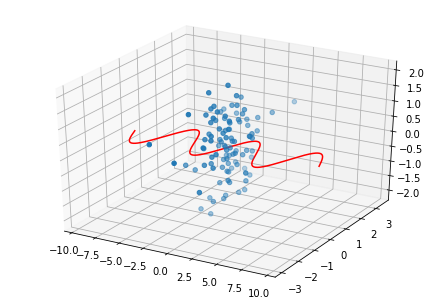### 三维子图

# 创建 1 张画布
fig = plt.figure(figsize=(8, 4))

# 向画布添加子图 1
ax1 = fig.add_subplot(1, 2, 1, projection='3d')
# 生成子图 1 数据
x = np.linspace(-6 * np.pi, 6 * np.pi, 1000)
y = np.sin(x)
z = np.cos(x)
# 绘制第 1 张图
ax1.plot(x, y, z)

# 向画布添加子图 2
ax2 = fig.add_subplot(1, 2, 2, projection='3d')
# 生成子图 2 数据
X = np.arange(-2, 2, 0.1)
Y = np.arange(-2, 2, 0.1)
X, Y = np.meshgrid(X, Y)
Z = np.sqrt(X ** 2 + Y ** 2)
# 绘制第 2 张图
ax2.plot_surface(X, Y, Z, cmap=plt.cm.winter)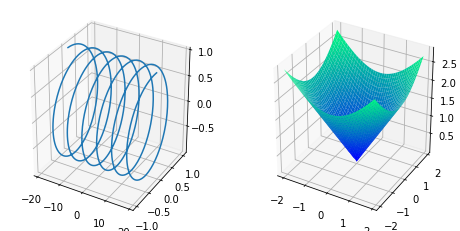#### 在线学习•